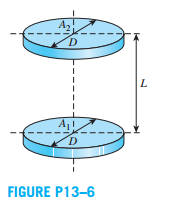Create an Account

Home / Questions / Consider two coaxial parallel circular disks of equal diameter D that are spaced apart by ...

Consider two coaxial parallel circular disks of equal diameter D that are spaced apart by a distance L If the view factor is

Consider two coaxial parallel circular disks of equal diameter D that are spaced apart by a distance L. If the view factor is F12 =  0.1, without altering the diameter of the disks, determine a solution that would increase the view factor F12 = 0.1 by a factor of 5.Jul 23 2020 View more View LessSubscribe To Get Solution